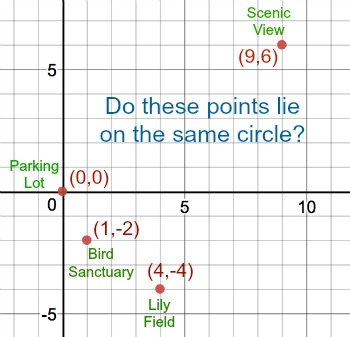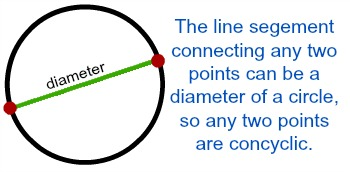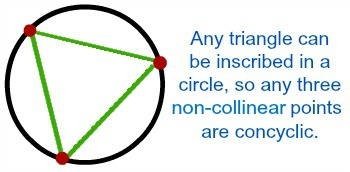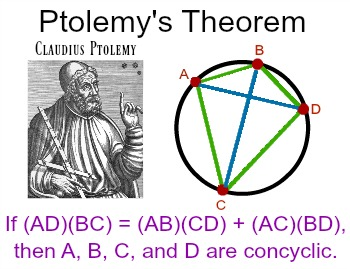# Concyclic Points: Definition & Proofs

Lesson Transcript
Instructor: Laura Pennington

Laura received her Master's degree in Pure Mathematics from Michigan State University, and her Bachelor's degree in Mathematics from Grand Valley State University. She has 20 years of experience teaching collegiate mathematics at various institutions.

Concyclic points are points that all lie on the same circle. This lesson will use some useful theorems to explain how to prove whether or not a set of two, three, or four points are concyclic. Updated: 05/25/2019

## Concyclic Points

Suppose you're at a park and there are three attractions you want to see. If you place the map of the park on a grid and let your starting point (the parking lot) be at the point (0,0), then your starting point and the attractions fall at the following points:

(0,0) (1,-2) (4,-4) (9,6)

It's a beautiful day, so you want to know if it's possible to walk a longer circular path that will visit all of the attractions rather than walking straight to each attraction. In other words, you want to know if there's a circle that all four points lie on.In mathematics, we call points that lie on the same circle, and are therefore the same distance from the center of the circle, concyclic points. Therefore, mathematically speaking, we want to know if the points (0,0), (1,-2), (4,-4), and (9,6) are concyclic points. Let's explore!

An error occurred trying to load this video.

Try refreshing the page, or contact customer support.

Coming up next: How Randomized Experiments Are Designed

### You're on a roll. Keep up the good work!

Replay
Your next lesson will play in 10 seconds
• 0:04 Concyclic Points
• 0:51 Proving Concyclic Points
• 2:47 Example
• 3:53 Lesson Summary
Save Save

Want to watch this again later?

Timeline
Autoplay
Autoplay
Speed Speed

## Proving Concyclic Points

Proving that a set of given points are concyclic sounds harder than it really is, depending on how many points are in the set. We have some really nice theorems to help us determine if a set of 2, 3, or 4 points are concyclic.

It's pretty easy to determine that any two points are concyclic. To illustrate this, consider the fact that if we draw a line between any two points, that line can be a diameter of a circle, and both of its endpoints would lie on a circle. Therefore, any two points are concyclic.When it comes to a set of three points, we have a nice theorem that allows us to determine (or prove) whether or not the three points are concyclic. That theorem states the following:

• Theorem: Any three points that are non-collinear (meaning they don't lie on the same line) are concyclic.

This is because if we connect any three non-collinear points with line segments, we form a triangle, and all triangles can be inscribed in a circle.Therefore, given a set of 3 points, we can prove they are concyclic by determining that they don't all lie on the same line.

This is great so far, but what we're most interested in is our four points in the park and whether or not they are concyclic. Thankfully, a Greek writer and mathematician, Claudius Ptolemy, created a theorem that makes proving that four points are concyclic much easier than one would expect.

• Ptolemy's Theorem: A quadrilateral can be drawn in a circle if and only if the product of the measures of its diagonals are equal to the sum of the products of the measures of the pairs of opposite sides.Since any four points determine a quadrilateral, we can use Ptolemy's Theorem to determine, or prove, that any four points are concyclic by:

1. Finding the product of the lengths of the diagonals of the quadrilateral formed by the points.
2. Finding the sum of the products of the measures of the pairs of opposite sides of the quadrilateral formed by the points.
3. If these two values are equal, the points are concyclic. If they're not equal, then the points are not concyclic.

To unlock this lesson you must be a Study.com Member.

### Register to view this lesson

Are you a student or a teacher?